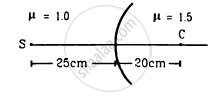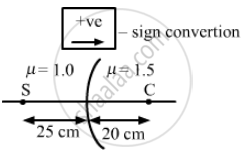Advertisement Remove all ads

# P Locate the Image Formed by Refraction in the Situation Shown in Figure. - Physics

Sum

Locate the image formed by refraction in the situation shown in figure.Advertisement Remove all ads

#### Solution

Given,
Let the refractive indices of two mediums be μ1=1.0 and μ2 =1.5
Point C is the centre of curvature, the distance between C and the pole is 20 cm.
Therefore, radius of curvature (R) = 20 cm
Distance between source S and pole is 25 cm.
Therefore, object distance (u) = −25
$\frac{\mu_2}{v} - \frac{\mu_1}{u} = \frac{\mu_2 - \mu_1}{R}$$\Rightarrow \frac{1 . 5}{v} - \frac{1}{( - 25)} = \frac{0 . 5}{20}$
$\Rightarrow \frac{1 . 5}{v} = \frac{- 3}{200}$
$\therefore v = - \frac{200 \times 1 . 5}{0 . 3 \times 10} = - 100$
Hence, the required location of the image is 100 cm from the pole and on the side of S.

Concept: Refraction
Is there an error in this question or solution?
Advertisement Remove all ads

#### APPEARS IN

HC Verma Class 11, 12 Concepts of Physics 1
Chapter 18 Geometrical Optics
Q 37 | Page 414
Advertisement Remove all ads

#### Video TutorialsVIEW ALL 

Advertisement Remove all ads
Share
Notifications

View all notifications

Forgot password?